# 后缀树

## 一些记号表示中的第个字符，其中表示中第个字符至第个字符组成的字符串，称为的一个子串。的以开头的后缀，的以结尾的前缀。

## 定义## 后缀树的建立

### 支持前端动态添加字符的算法

  1 2 3 4 5 6 7 8 9 10 11 12 13 14 15 16 17 18 19 20 21 22 23 24 25 26 27 28 29 30 31 32 33 struct SuffixAutomaton { int tot, lst; int siz[N << 1]; int buc[N], id[N << 1]; struct Node { int len, link; int ch; } st[N << 1]; SuffixAutomaton() : tot(1), lst(1) {} void extend(int ch) { int cur = ++tot, p = lst; lst = cur; siz[cur] = 1, st[cur].len = st[p].len + 1; for (; p && !st[p].ch[ch]; p = st[p].link) st[p].ch[ch] = cur; if (!p) st[cur].link = 1; else { int q = st[p].ch[ch]; if (st[q].len == st[p].len + 1) st[cur].link = q; else { int pp = ++tot; st[pp] = st[q]; st[pp].len = st[p].len + 1; st[cur].link = st[q].link = pp; for (; p && st[p].ch[ch] == q; p = st[p].link) st[p].ch[ch] = pp; } } } } SAM; 

### 支持后端动态添加字符的算法

Ukkonen 算法是一种增量构造算法。我们依次向树中插入串的每一个字符，并在每一次插入之后正确地维护当前的后缀树。

#### 朴素算法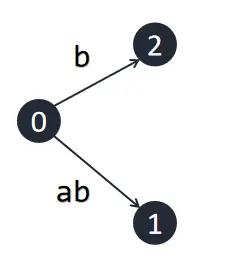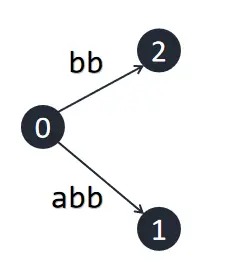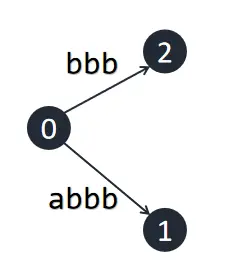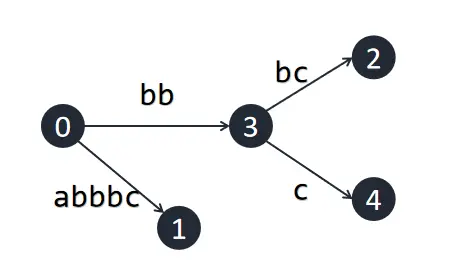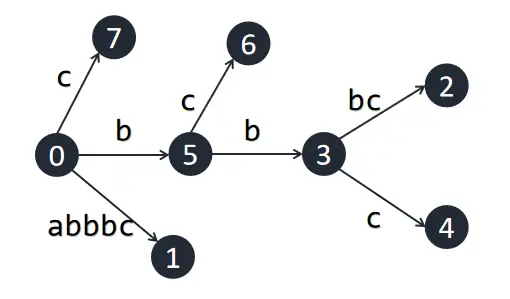#### Ukkonen 算法

Ukkonen 算法的整体流程如下：

1.位置已经存在的转移。此时后缀树形态不会发生变化。由于已经在后缀树中出现，所以对于也会在后缀树中出现，此时只需将，不需做任何修改。
2.不存在的转移。如果恰好为树中的节点，则此节点新增一条出边；否则需要对节点进行分裂，在此位置新增一个节点，并在新增节处添加出边。此时对于，我们并不知道会对后缀树形态造成什么影响，所以我们还需继续考虑。考虑怎么求出在后缀树中的位置：如果不为，可以利用后缀链接，令；否则，令。最后令，再次重复这个过程。

  1 2 3 4 5 6 7 8 9 10 11 12 13 14 15 16 17 18 19 20 21 22 23 24 25 26 27 28 29 30 31 32 33 34 35 36 37 38 39 40 41 42 struct SuffixTree { int ch[M + 5][RNG + 1], st[M + 5], len[M + 5], link[M + 5]; int s[N + 5]; int now{1}, rem{0}, n{0}, tot{1}; SuffixTree() { len = inf; } int new_node(int s, int le) { ++tot; st[tot] = s; len[tot] = le; return tot; } void extend(int x) { s[++n] = x; ++rem; for (int lst{1}; rem;) { while (rem > len[ch[now][s[n - rem + 1]]]) rem -= len[now = ch[now][s[n - rem + 1]]]; int &v{ch[now][s[n - rem + 1]]}, c{s[st[v] + rem - 1]}; if (!v || x == c) { lst = link[lst] = now; if (!v) v = new_node(n, inf); else break; } else { int u{new_node(st[v], rem - 1)}; ch[u][c] = v; ch[u][x] = new_node(n, inf); st[v] += rem - 1; len[v] -= rem - 1; lst = link[lst] = v = u; } if (now == 1) --rem; else now = link[now]; } } } Tree; 

## 例题

### 洛谷 P3804【模板】后缀自动机（SAM）

  1 2 3 4 5 6 7 8 9 10 11 12 13 14 15 16 17 18 19 20 21 22 23 24 25 26 27 28 29 30 31 32 33 34 35 36 37 38 39 40 41 42 43 44 45 46 47 48 49 50 51 52 53 54 55 56 57 58 59 60 61 62 63 64 65 66 67 68 69 70 71 #include using namespace std; constexpr int N(1e6), M(2 * N), inf(1e7), RNG{26}; struct SuffixTree { int ch[M + 5][RNG + 1], st[M + 5], len[M + 5], link[M + 5]; int s[N + 5]; int now{1}, rem{0}, n{0}, tot{1}; SuffixTree() { len = inf; } int new_node(int s, int le) { ++tot; st[tot] = s; len[tot] = le; return tot; } void extend(int x) { s[++n] = x; ++rem; for (int lst{1}; rem;) { while (rem > len[ch[now][s[n - rem + 1]]]) rem -= len[now = ch[now][s[n - rem + 1]]]; int &v{ch[now][s[n - rem + 1]]}, c{s[st[v] + rem - 1]}; if (!v || x == c) { lst = link[lst] = now; if (!v) v = new_node(n, inf); else break; } else { int u{new_node(st[v], rem - 1)}; ch[u][c] = v; ch[u][x] = new_node(n, inf); st[v] += rem - 1; len[v] -= rem - 1; lst = link[lst] = v = u; } if (now == 1) --rem; else now = link[now]; } } pair search(int u, int dep = 0) { if (st[u] + len[u] >= n) return {0, 1}; dep += len[u]; long long ans{0}; int ys{0}; for (int i{0}; i <= RNG; ++i) if (ch[u][i]) { auto [__, _]{search(ch[u][i], dep)}; ans = max(ans, __); ys += _; } if (ys > 1) ans = max(ans, 1LL * dep * ys); return {ans, ys}; } } T; char s[N + 5]; int main() { scanf("%s", s + 1); for (int i{1}; s[i]; ++i) T.extend(s[i] - 'a' + 1); T.extend(0); cout << T.search(1).first << endl; return 0; } 

### CF235C Cyclical Quest

  1 2 3 4 5 6 7 8 9 10 11 12 13 14 15 16 17 18 19 20 21 22 23 24 25 26 27 28 29 30 31 32 33 34 35 36 37 38 39 40 41 42 43 44 45 46 47 48 49 50 51 52 53 54 55 56 57 58 59 60 61 62 63 64 65 66 67 68 69 70 71 72 73 74 75 76 77 78 79 80 81 82 83 84 85 86 87 88 89 90 91 92 93 94 95 96 97 98 99 100 101 102 103 104 105 106 107 #include using namespace std; constexpr int N(1e6); struct SuffixTree { static constexpr int N{2 * ::N}, RNG{26}, inf = 1e7; int ch[N + 5][RNG + 1]; int st[N + 5], len[N + 5], link[N + 5], s[::N + 5]; int now{1}, rem{0}, tot{1}, n{0}; int cnt[N + 5], vis[N + 5]; SuffixTree() { len = inf; } void clear() { memset(ch, 0, sizeof ch); now = tot = 1; rem = n = 0; } int new_node(int s, int le) { ++tot; st[tot] = s; len[tot] = le; return tot; } void extend(int x) { s[++n] = x; ++rem; for (int lst{1}; rem;) { while (rem > len[ch[now][s[n - rem + 1]]]) rem -= len[now = ch[now][s[n - rem + 1]]]; int &v{ch[now][s[n - rem + 1]]}, c{s[st[v] + rem - 1]}; if (!v || x == c) { lst = link[lst] = now; if (!v) v = new_node(n, inf); else break; } else { int u{new_node(st[v], rem - 1)}; ch[u][c] = v; ch[u][x] = new_node(n, inf); st[v] += rem - 1; len[v] -= rem - 1; lst = link[lst] = v = u; } if (now == 1) --rem; else now = link[now]; } } void init(int u) { if (len[u] > 1e6) return cnt[u] = 1, void(); for (int i{0}; i <= RNG; ++i) if (ch[u][i]) init(ch[u][i]), cnt[u] += cnt[ch[u][i]]; } long long test(const char *t, int m) { static int time{0}; ++time; int now{1}, rem{0}, o{0}; long long ans{0}; for (int i{1}; i <= m; ++i) { while (o < i + m - 1) { while (rem >= len[ch[now][t[o - rem + 1]]]) rem -= len[now = ch[now][t[o - rem + 1]]]; int v{ch[now][t[o - rem + 1]]}, c{s[st[v] + rem]}; if (v && c == t[o + 1]) { ++o; ++rem; } else { break; } } if (o == i + m - 1 && vis[ch[now][t[o - rem + 1]]] != time) ans += cnt[ch[now][t[o - rem + 1]]], vis[ch[now][t[o - rem + 1]]] = time; if (now == 1) --rem; else now = link[now]; } return ans; } } T; char s[N * 2 + 5]; int main() { scanf("%s", s + 1); for (int i{1}; s[i]; ++i) T.extend(s[i] - 'a' + 1); T.extend(0); T.init(1); int pw; cin >> pw; while (pw--) { scanf("%s", s + 1); int n{strlen(s + 1)}; for (int i{1}; i <= n; ++i) s[i] += 1 - 'a'; copy(s + 1, s + 1 + n, s + 1 + n); cout << T.test(s, n) << "\n"; } return 0; } 

## 参考文献

1. 2021 国家集训队论文《后缀树的构建》代晨昕
2. 炫酷后缀树魔术 - EternalAlexander 的博客